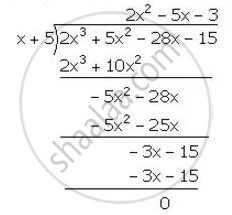Share

Books Shortlist

# (X + 5) is a Factor of 2x3 + 5x2 – 28x – 15. Hence, Factorise the Expression 2x3 + 5x2 – 28x – 15 Completely. - ICSE Class 10 - Mathematics

#### Question

(x + 5) is a factor of 2x3 + 5x2 – 28x – 15. Hence, factorise the expression 2x3 + 5x2 – 28x – 15 completely.

#### Solution

Let f(x)=2x^3+5x^2-28x-15

x+5=0  ⇒ x=-5

∴ Remainder = f(-5)

=2(-5)^3+5(-5)^2-28(-5)-15

=-250+125+140-15

=-265+265

=0

Hence, (x+5)is a factoor of f(x)

Now, we have,∴ 2x^3+5x^2-28x-15=(x+5) (2x^2-5x-3)

=(x+5)[2x^2+6x+x-3]

=(x-5)[2x(x-3)+1(x-3)]

=(x+5) (2x+1) (x-3)

Is there an error in this question or solution?

#### Video TutorialsVIEW ALL 

Solution (X + 5) is a Factor of 2x3 + 5x2 – 28x – 15. Hence, Factorise the Expression 2x3 + 5x2 – 28x – 15 Completely. Concept: Factor Theorem.
S